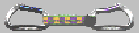# Approximation, Simplification and Smoothing of Polygonal Curves

Piecewise curvilinear approximation of planar curves is a useful technique in many engineering applications. The general goal is to represent a curve by a sequence of circular arcs and straight-line segments, within a given tolerance. The advantage of such an approximation is that it allows a curve to be represented by a (typically) comparatively small number of arcs and line segments, thus achieving a noise reduction and data compression.

From a practical point of view, it is particularly desirable to achieve tangent-continuous approximations: NC machining as well as rapid prototyping benefit from the availability of so-called G1 curves. In particular, high-speed machining greatly depends on smooth tool paths.

As part of Eibl's diploma (MSc) thesis, Johannes Eibl and Martin Held worked on the approximation of polygonal curves by biarc curves. Given a simple (planar) polygon, our biarc approximation algorithm finds a tangent-continuous approximation curve A that consists of biarcs (and straight-line segments). The approximation curve A is required to lie within a specified tolerance zone relative to P, thus guaranteeing a bounded maximum deviation of A from P. If requested by the user, the algorithm can also guarantee that P lies in a tolerance zone relative to A.

We studied both symmetric and asymmetric tolerance zones. In particular, the tolerance zone may lie completely at one side of P, e.g., in the interior of P. Dealing with asymmetric or one-sided tolerances is of importance for applications which do not tolerate approximation errors on both sides of P. For instance, in NC machining it is often preferred to drive the tool such that any approximation error results in under-cutting rather than in over-cutting.

Our approximation algorithm also guarantees the approximation curve A to be simple. In particular, the algorithm shrinks the tolerance appropriately at constrictions of P. Thus, the approximation curve A does not contain any self-intersections, and is globally valid.

later on, Martin Heimlich and I designed a second approximation algorithm that relies on the Voronoi diagram of the input for computing (what we call) tolerance bands as subsets of the tolerance zones. We also added support for additional approximation primitives: our new algorithm can approximate sets of closed simple polygons by straight-line segments, circular biarcs, elliptic arcs, elliptic biarcs, and cubic Bezier curves. (That is, this algorithm can also be used for transforming an N-vertex polygon into an M-vertex polygon such that N<<M and approximation tolerances are met.) Of course, also our new algorithm maintains the topology of the input and can handle asymmetric or even one-sided tolerances.

Extensive experiments with synthetic and real-world data sets show that this new algorithm generates approximation curves with significantly fewer approximation primitives than previously proposed algorithms, including the t-part based approach of Eibl and myself. This difference becomes the more prominent the larger the tolerance threshold is or the more severe the noise in the input is. In particular, no heuristic is needed for smoothing noisy input prior to the actual approximation. Rather, our approximation algorithm can be used to smooth out noise in a reliable manner.

More recently, Dominik Kaaser and I introduced an efficient algorithm for computing C2 approximations of a set of planar curvilinear profiles by means of uniform cubic B-splines. The resulting approximations are guaranteed to lie within a user-specified tolerance of the input profiles, with asymmetric and even one-sided tolerances being supported by our algorithm. Furthermore, as in the work with Martin Heimlich, the input topology is reflected by our approximation. The input profiles may consist of straight-line segments and circular arcs.

We use a top-down fitting scheme to generate a (hopefully) small number of B-spline segments. This allows our approximation algorithm to run in O(N log N) time and O(N) space, where N denotes the number of input segments and arcs that form the profiles. Extensive experiments with synthetic and real-world data sets show that our algorithm works very nicely in practice. In particular, it supports the approximation of profiles with up to \$10\,000\$ input segments and arcs in less than ten seconds on a standard PC.

Work on this project was supported by the Austrian Science Foundation (FWF) within FWF Project L43-N12.

Related publications:

M. Held, D. Kaaser (2014):
"C2 Approximation of Planar Curvilinear Profiles by Cubic B-Splines".
Computer-Aided Design and Applications, 11(2):206-219, 2014.

M. Held, D. Kaaser (2013):
"Curvature-Continuous Approximation of Planar Curvilinear Profiles".
Proc. 10th Annual CAD Conference, p. 90-91, Bergamo, Italy, June 2013.

M. Heimlich, M. Held (2008):
"Biarc Approximation, Simplification and Smoothing of Polygonal Curves by Means of Voronoi-based Tolerance Bands".
Int. J. of Computational Geometry and Applications, 18(3):221--250, June 2008.

M. Held, J. Eibl (2005):
"Biarc Approximation of Polygons Within Asymmetric Tolerance Bands".
Computer-Aided Design, 37(4): 357--371, April 2005.

J. Eibl, M. Held (2002):
"On a Simple Algorithm for Approximating Polygons by Biarc Curves Within Asymmetric Tolerance Bands".
SoCGMA'02, Vienna, Austria.

Our biarc approximation code was tested on hundreds of synthetic and real-world data sets. The following images show biarc approximations which were computed by means of our code. (Click on an image icon in order to see the full-size image. The full-size images have roughly 800x800 pixels.)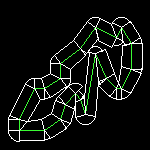Symmetric biarc approximation of a polygon: the image on the left shows the input polygon (in green) and the symmetric tolerance band (in white) for an approximation tolerance of roughly 0.1 units, and the image on the right shows the biarc approximation computed.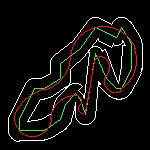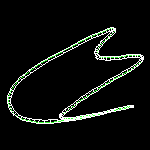Asymmetric biarc approximation of a polygon: the image on the left shows the input polygon (in green) and the asymmetric tolerance band (in white) for an approximation tolerance of roughly 0.01 units, and the image on the right shows the biarc approximation computed.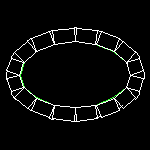One-sided biarc approximation of an "ellipse" polygon: the image on the left shows the input polygon (in green) and the one-sided tolerance band (in white) for an approximation tolerance of roughly 0.1 units, and the image on the right shows the biarc approximation computed.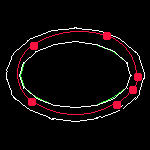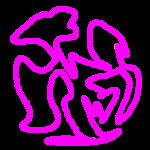Symmetric biarc approximation of a 1024-gon: the image on the left shows the input polygon (in green) and the vertices of the input polygon (in magenta), and the image on the right shows the biarc approximation computed for an approximation tolerance of roughly 0.002 units.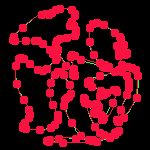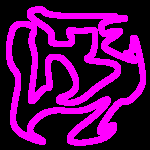Symmetric biarc approximation of a 32768-gon: the image on the left shows the input polygon (in green) and the vertices of the input polygon (in magenta), and the image on the right shows the biarc approximation computed for an approximation tolerance of roughly 0.00006 units.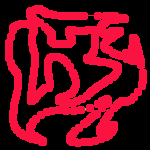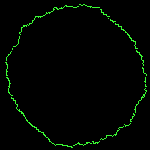Symmetric biarc approximation of a noisy polygonal model of a circle: the image on the left shows the input polygon (in green), and the image on the right shows the biarc approximation computed after polygonal smoothing of the data by the biarc code for two times; the tolerance band corresponding to the second smoothing is depicted in white. Note that the resulting biarc approximation still respects the user's approximation tolerance relative to the original input! (For visual purposes both the jaggedness and the approximation tolerance have been exaggerated compared to typical values that oocur for real-world data.)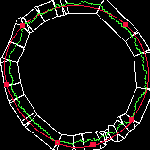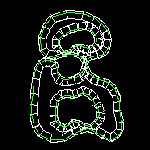Simultaneous symmetric biarc approximation of a three polygons: the image on the left shows the input polygon (in green) and the resulting tolerance bands (in white), and the image on the right shows the biarc approximation computed. Note that all three biarc curves are simple and do not intersect each other!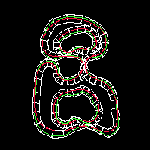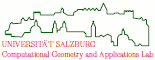file last modified: Wednesday, 20-Oct-2021 08:04:27 CESTCopyright © 2022 Martin Held. All rights reserved.